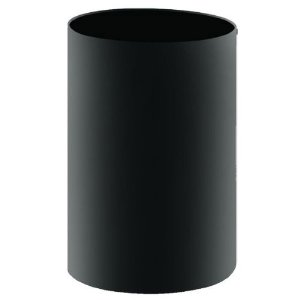# Ratio of surface areas

Geometry Level 2

The total surface area and the curved surface area of a cylinder that is open at the top are in the ratio $3:1$. What is the ratio of the radius of the cylinder to that of the height?

If your answer is the form of $a: b$, where $a$ and $b$ are coprime positive integers, find $a+b$.×